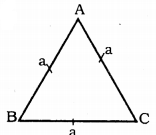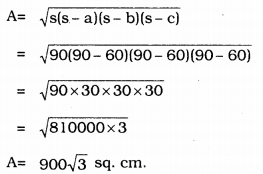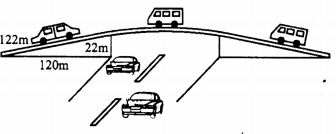# KSEEB Solutions for Class 9 Maths Chapter 8 Heron’s Formula Ex 8.1

KSEEB Solutions for Class 9 Maths Chapter 8 Heron’s Formula Ex 8.1 are part of KSEEB Solutions for Class 9 Maths. Here we have given Karnataka Board Class 9 Maths Chapter 8 Heron’s Formula Exercise 8.1.

## Karnataka Board Class 9 Maths Chapter 8 Heron’s Formula Ex 8.1

Memorizing class 10 maths formulas is highly crucial for students to score better in the board exam as well as entrance exams.

Question 1.
A traffic signal board, indicating ‘SCHOOL AHEAD’, is an equilateral triangle with side ‘a’. Find the area of the signal board, using Heron’s formula. If its perimeter is 180 cm, what will be the area of the signal board ?
Solution:
Let each side of the signal board be ‘a’ unit.Its’ perimeter,
AB + BC + CA = 180 cm
Area = ?
Perimeter of ∆ABC
a + a + a = 180
∴ 3a = 180
∴ a = $$\frac{180}{3}$$
∴ a = 60 cm
a = b = c = 60 cm
$$s=\frac{a+b+c}{2}=\frac{180}{2}=90$$
Area of ∆ABC A,Question 2.
The triangular side walls of a flyover have been used for advertisements. The sides of the walls are 122 m, 22 m and 120 m. The advertisements yield an earning of Rs. 5000 per m2 per year. A company hired one of its walls for 3 months. How much rent did it pay?Solution:
Sides of triangle, a = 122m, b = 22m, c = 120m.Rent for each year, each sq.m. = Rs. 5,000
Rent for each month, each sq.m. = $$\frac{5000}{12}$$
Rent for each quarterly, each sq.m. = 3 × $$\frac{5000}{12}$$
= Rs. 1250
∴ Rent for 1320 sq.m.= 1250 × 1320 = Rs. 1650000
∴ Amount company has to pay is Rs. 1650000.

Question 3.
There is a slide in a park. One of its side walls has been painted in some colour with a message “KEEP THE PARK GREEN AND CLEAN”. If the sides of the wall are 15m, 11m and 6 m, find,the area painted in colour.Solution:
Sides, a = 15 m, b = 11 m, c = 6 m.Question 4.
Find the area of a triangle two sides of which are 18 cm and 10 cm and the perimeter is 42 cm.
Solution:
Three sides of triangle be
Let a = 18 cm, b = 10 cm, c = x cm.
Perimeter = 42 cm.
a + b + c = 42
18 + 10 + x = 42
28 + x = 42
∴ x = 42 – 28
∴ x = 14 cm
∴ c = 14 cmQuestion 5.
Sides of a triangle are in the ratio of 12: 17: 25 and its perimeter is 540 cm. Find its area.
Solution:
Let sides of triangle be
a = 12x, b = 17x and c = 25x
∴ Perimeter = 12x + 17x + 25x = 540
54x = 540
∴ x = $$\frac{540}{54}$$
∴ x = 10 cm.
∴ a = 12x = 12 × 10 = 120 cm.
b = 17x = 17 × 10 = 170 cm.
c = 25x = 25 × 10 = 250 cm.Question 6.
An isosceles triangle has perimeter 30 cm and each of the equal sides is 12 cm. Find the area of the triangle.
Solution:
Let the sides of isosceles triangle be a and b.
∴ a = b = 12 cm.
Let the third side be ‘c’.
∴ Perimeter = a + b + c = 30
= 12 + 12 + c = 30
24 + c = 30
c = 30 – 24
c = 6 cm.We hope the KSEEB Solutions for Class 9 Maths Chapter 8 Heron’s Formula Ex 8.1 help you. If you have any query regarding Karnataka Board Class 9 Maths Chapter 8 Heron’s Formula Exercise 8.1, drop a comment below and we will get back to you at the earliest.

error: Content is protected !!